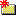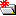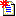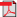Products Download Events Support Videos All Product Families ARM7, ARM9, and Cortex-M3 Products C16x, XC16x, and ST10 Products C251 and 80C251 Products Cx51 and 8051 Products Modified Anytime In the Last 7 Days In the Last 14 Days In the Last 30 Days In the Last 90 Days In the Last 6 Months

Technical Support

On-Line Manuals

Compiler Reference GuidePrefaceArm Compiler Tools Overviewarmclang Referencearmlink Referencefromelf Referencearmar Referencearmasm Legacy Assembler ReferenceAppendixesStandard C Implementation DefinitionImplementation definitionTranslationTranslation limitsEnvironmentIdentifiersCharactersIntegersFloating-pointArrays and pointersHintsStructures, unions, enumerations, and bitfieldsQualifiersPreprocessing directivesLibrary functionsArchitectureStandard C++ Implementation DefinitionImplementation definitionGeneralLexical conventionsBasic conceptsStandard conversionsExpressionsDeclarationsDeclaratorsTemplatesException handlingPreprocessing directivesLibrary introductionLanguage support libraryGeneral utilities libraryStrings libraryNumerics libraryLocalization libraryContainers libraryInput/output libraryRegular expressions libraryAtomic operations libraryThread support libraryImplementation quantitiesVia File SyntaxOverview of via filesVia file syntax rulesArm Compiler Reference Guide ChangesChanges for the Arm Compiler Reference Guide

# <!-- var kmNextPage = "armclang_ref_vuz1493281532660.htm"; var kmPrevPage = "armclang_ref_lin1493281485740.htm"; var kmNextPageTitle = "Architecture"; var kmPrevPageTitle = "Preprocessing directives"; var kmBreadCrumbs = "<a href=\"default.htm\">Home</a> &raquo; <a href=\"armclang_ref_fyk1564498919356.htm\">Appendixes</a> &raquo; <a href=\"armclang_ref_pvk1493280214273.htm\">Standard C Implementation Definition</a> &raquo; Library functions"; kmSetupPaging (); kmNavButtons(); //--> Library functions

## A.14 Library functions

Describes implementation-defined aspects of the Arm® C compiler and C library relating to library functions, as required by the ISO C standard.

Any library facilities available to a freestanding program, other than the minimal set required by clause 4 (5.1.2.1).
The Arm Compiler provides the Arm C Micro-library. For information about facilities, provided by this library, see The Arm® C Micro-library in the Arm® C and C++ Libraries and Floating-Point Support User Guide.
The format of the diagnostic printed by the assert macro (7.2.1.1).
The assert macro prints a diagnostic in the format:
`*** assertion failed: `expression`, `filename`, `line number``
The representation of the floating-points status flags stored by the `fegetexceptflag` function (7.6.2.2).
The `fegetexceptflag` function stores the floating-point status flags as a bit set as follows:
• Bit 0 (`0x01`) is for the Invalid Operation exception.
• Bit 1 (`0x02`) is for the Divide by Zero exception.
• Bit 2 (`0x04`) is for the Overflow exception.
• Bit 3 (`0x08`) is for the Underflow exception.
• Bit 4 (`0x10`) is for the Inexact Result exception.
Whether the feraiseexcept function raises the Inexact floating-point exception in addition to the Overflow or Underflow floating-point exception (7.6.2.3).
The `feraiseexcept` function does not raise by itself the Inexact floating-point exception when it raises either an Overflow or Underflow exception.
Strings other than `"C"` and `""` that can be passed as the second argument to the `setlocale` function (7.11.1.1).
What other strings can be passed as the second argument to the `setlocale` function depends on which `__use_X_ctype` symbol is imported (`__use_iso8859_ctype`, `__use_sjis_ctype`, or `__use_utf8_ctype`), and on user-defined locales.
The types defined for `float_t` and `double_t` when the value of the `FLT_EVAL_METHOD` macro is less than 0 (7.12).
The types defined for `float_t` and `double_t` are float and double, respectively, for all the supported values of `FLT_EVAL_METHOD`.
Domain errors for the mathematics functions, other than those required by this International Standard (7.12.1).
The following functions return additional domain errors under the specified conditions (the function name refers to all the variants of the function. For example, the `acos` entry applies to `acos`, `ascof`, and `acosl` functions):
Function Condition Return value Error
`acos(x)` `abs(x) > 1` `NaN` `EDOM`
`asin(x)` `abs(x) > 1` `NaN` `EDOM`
`cos(x)` `X == Inf` `NaN` `EDOM`
`sin(x)` `x == Inf` `NaN` `EDOM`
`tan(x)` `x == Inf` `NaN` `EDOM`
`atanh(x)` `abs(x) == 1` `Inf` `ERANGE`
`ilogb(x)` `x == 0.0` `-INT_MAX` `EDOM`
`ilogb(x)` `x == Inf` `INT_MAX` `EDOM`
`ilogb(x)` `x == NaN` `FP_ILOGBNAN` `EDOM`
`log(x)` `x < 0` `NaN` `EDOM`
`log(x)` `x == 0` `-Inf` `ERANGE`
`log10(x)` `x < 0` `NaN` `EDOM`
`log10(x)` `x == 0` `-Inf` `ERANGE`
`log1p(x)` `x < -1` `NaN` `EDOM`
`log1p(x)` `x == -1` `-Inf` `ERANGE`
`log2(x)` `x < 0` `NaN` `EDOM`
`log2(x)` `x == 0` `-Inf` `ERANGE`
`logb(x)` `x == 0` `-Inf` `EDOM`
`logb(x)` `x == Inf` `+Inf` `EDOM`
`pow(x, y)` `y < 0` and (`x == +0` or `y` is even) `+Inf` `ERANGE`
`pow(x, y)` `y < 0` and `x == -0` and `y` is odd `-Inf` `ERANGE`
`pow(x, y)` `y < 0` and `x == -0` and `y` is non-integer `+Inf` `ERANGE`
`pow(x,y)` `x < 0` and `y` is non-integer `NaN` `EDOM`
`sqrt(x)` `x < 0` `NaN` `EDOM`
`lgamma(x)` `x <= 0` `Inf` `ERANGE`
`tgamma(x)` `x < 0` and `x` is integer `NaN` `EDOM`
`tgamma(x)` `x == 0` `Inf` `ERANGE`
`fmod(x,y)` `x == Inf` `NaN` `EDOM`
`fmod(x,y)` `y == 0` `NaN` `EDOM`
`remainder(x, y)` `y == 0` `NaN` `EDOM`
`remquo(x, y, q)` `y == 0` `NaN` `EDOM`
The values returned by the mathematics functions on domain errors or pole errors (7.12.1).
See previous table.
The values returned by the mathematics functions on underflow range errors, whether `errno` is set to the value of the macro `ERANGE` when the integer expression `math_errhandling & MATH_ERRNO` is nonzero, and whether the Underflow floating-point exception is raised when the integer expression `math_errhandling & MATH_ERREXCEPT` is nonzero. (7.12.1).
On underflow, the mathematics functions return `0.0`, the `errno` is set to `ERANGE`, and the Underflow and Inexact exceptions are raised.
Whether a domain error occurs or zero is returned when an fmod function has a second argument of zero (7.12.10.1).
When the second argument of `fmod` is zero, a domain error occurs.
Whether a domain error occurs or zero is returned when a remainder function has a second argument of zero (7.12.10.2).
When the second argument of the remainder function is zero, a domain error occurs and the function returns NaN.
The base-2 logarithm of the modulus used by the remquo functions in reducing the quotient (7.12.10.3).
The base-2 logarithm of the modulus used by the `remquo` functions in reducing the quotient is 4.
Whether a domain error occurs or zero is returned when a remquo function has a second argument of zero (7.12.10.3).
When the second argument of the `remquo` function is zero, a domain error occurs.
Whether the equivalent of `signal(sig, SIG_DFL);` is executed prior to the call of a signal handler, and, if not, the blocking of signals that is performed (7.14.1.1).
The equivalent of `signal(sig, SIG_DFL)` is executed before the call to a signal handler.
The null pointer constant to which the macro NULL expands (7.19).
The macro `NULL` expands to 0.
Whether the last line of a text stream requires a terminating new-line character (7.21.2).
The last line of text stream does not require a terminating new-line character.
Whether space characters that are written out to a text stream immediately before a new-line character appear when read in (7.21.2).
Space characters, written out to a text stream immediately before a new-line character, appear when read back.
The number of null characters that may be appended to data written to a binary stream (7.21.2).
No null characters are appended at the end of a binary stream.
Whether the file position indicator of an append-mode stream is initially positioned at the beginning or end of the file (7.21.3).
The file position indicator of an append-mode stream is positioned initially at the end of the file.
Whether a write on a text stream causes the associated file to be truncated beyond that point (7.21.3).
A write to a text stream causes the associated file to be truncated beyond the point where the write occurred if this is the behavior of the device category of the file.
The characteristics of file buffering (7.21.3).
The C Library supports unbuffered, fully buffered, and line buffered streams.
Whether a zero-length file actually exists (7.21.3).
A zero-length file exists, even if no characters are written by an output stream.
The rules for composing valid file names (7.21.3).
Valid file names depend on the execution environment.
Whether the same file can be simultaneously open multiple times (7.21.3).
A file can be opened many times for reading, but only once for writing or updating.
The nature and choice of encodings used for multibyte characters in files (7.21.3).
The character input and output functions on wide-oriented streams interpret the multibyte characters in the associated files according to the current chosen locale.
The effect of the remove function on an open file (7.21.4.1).
Depends on the environment.
The effect if a file with the new name exists prior to a call to the rename function (7.21.4.2).
Depends on the environment.
Whether an open temporary file is removed upon abnormal program termination (7.21.4.3).
Depends on the environment.
Which changes of mode are permitted (if any), and under what circumstances (7.21.5.4)
No changes of mode are permitted.
The style used to print an infinity or NaN, and the meaning of any n-char or n-wchar sequence printed for a NaN (7.21.6.1, 7.29.2.1).
A double argument to the `printf` family of functions, representing an infinity is converted to `[-]inf`. A double argument representing a NaN is converted to `[-]nan`. The F conversion specifier, produces `[-]INF` or `[-]NAN`, respectively.
The output for `%p` conversion in the `fprintf` or `fwprintf` function (7.21.6.1, 7.29.2.1).
The `fprintf` and `fwprintf` functions print %p arguments in lowercase hexadecimal format as if a precision of 8 (16 for 64-bit) had been specified. If the variant form (%#p) is used, the number is preceded by the character `@`.

### Note:

Using the `#` character with the `p` format specifier is undefined behavior in C11. armclang issues a warning.
The interpretation of a - character that is neither the first nor the last character, nor the second where a `^` character is the first, in the scanlist for `%[` conversion in the `fscanf` or `fwscanf` function (7.21.6.2, 7.29.2.1).
`fscanf` and `fwscanf` always treat the character `-` in a `%...[...]` argument as a literal character.
The set of sequences matched by a `%p` conversion and the interpretation of the corresponding input item in the `fscanf` or `fwscanf` function (7.21.6.2, 7.29.2.2).
`fscanf` and `fwscanf` treat `%p` arguments exactly the same as `%x` arguments.
The value to which the macro errno is set by the fgetpos, fsetpos, or ftell functions on failure (7.21.9.1, 7.21.9.3, 7.21.9.4).
On failure, the functions `fgetpos`, `fsetpos`, and `ftell` set the `errno` to `EDOM`.
The meaning of any n-char or n-wchar sequence in a string representing a NaN that is converted by the `strtod`, `strtof`, `strtold`, `wcstod`, `wcstof`, or `wcstold` function (7.22.1.3, 7.29.4.1.1).
Any n-char or n-wchar sequence in a string, representing a NaN, that is converted by the `strtod`, `strtof`, `strtold`, `wcstod`, `wcstof`, or `wcstold` functions, is ignored.
Whether or not the `strtod`, `strtof`, `strtold`, `wcstod`, `wcstof`, or `wcstold` function sets errno to `ERANGE` when underflow occurs (7.22.1.3, 7.29.4.1.1).

The `strtod`, `strtold`, `wcstod`, `wcstof`, or `wcstold` functions set `errno` to `ERANGE` when underflow occurs.

The strtof function sets the errno to `ERANGE` by default (equivalent to compiling with `-ffp-mode=std`) and doesn't, when compiling with `-ffp-mode=full` or `-fno-fast-math`.

Whether the `calloc`, `malloc`, and `realloc` functions return a null pointer or a pointer to an allocated object when the size requested is zero (7.22.3).

If the size of area requested is zero, `malloc()` and `calloc()` return a pointer to a zero-size block.

If the size of area requested is zero, `realloc()` returns `NULL`.

Whether open streams with unwritten buffered data are flushed, open streams are closed, or temporary files are removed when the abort or `_Exit` function is called (7.22.4.1, 7.22.4.5).

The function `_Exit` flushes the streams, closes all open files, and removes the temporary files.

The function `abort()` does not flush the streams and does not remove temporary files.

The termination status returned to the host environment by the `abort`, `exit`, `_Exit()`, or `quick_exit` function (7.22.4.1, 7.22.4.4, 7.22.4.5, 7.22.4.7).
The function `abort()` returns termination status 1 to the host environment. The functions `exit()` and `_Exit()` return the same value as the argument that was passed to them.
The value returned by the system function when its argument is not a null pointer (7.22.4.8).
The value returned by the system function when its argument is not a null pointer depends on the environment.
The range and precision of times representable in `clock_t` and `time_t` (7.27).
The types `clock_t` and `time_t` can represent integers in the range [0, 4294967295].
The local time zone and Daylight Saving Time (7.27.1).
Depends on the environment.
The era for the clock function (7.27.2.1).
Depends on the environment.
The `TIME_UTC` epoch (7.27.2.5).
`TIME_UTC` and `timespec_get` are not implemented.
The replacement string for the `%Z` specifier to the `strftime` and `wcsftime` functions in the `"C"` locale (7.27.3.5, 7.29.5.1).
The functions `strftime` and `wcsftime` replace `%Z` with an empty string.
Whether the functions in `<math.h>` honor the rounding direction mode in an IEC 60559 conformant implementation, unless explicitly specified otherwise (F.10).
Arm Compiler does not declare `__STDC_IEC_559__` and does not support Annex F of ISO/IEC 9899:2011.
 Non-ConfidentialPDF version 101754_0615_00_en Copyright © 2019, 2020 Arm Limited or its affiliates. All rights reserved.##### Important information

This site uses cookies to store information on your computer. By continuing to use our site, you consent to our cookies.